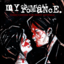MathematicsOpenStudy (anonymous):

Solve. Answers should be in Scientific Notation. (3.2 x 10^4) x (1.4 x 10^3) (8.4 x 10^8) / (2.2 x 10^2)OpenStudy (anonymous):

2.0OpenStudy (anonymous):

for the firstOpenStudy (anonymous):

Do you solve the actual notation before you compute, or is there a way to compute it without having to do all of that?OpenStudy (anonymous):

the actual notationOpenStudy (anonymous):

$(3.2 \times 10^4) \times (1.4 \times 10^3)$ $=(3.2\times 1.4)\times 10^{4+3}=4.48\times 10^7$OpenStudy (anonymous):

similarly, $(8.4 \times 10^8) \div (2.2 \times 10^2)=8.4\div 2.2\times 10^{8-2}=3.82\times 10^6$ but $\frac{8.4}{2.2}=\frac{84}{22}=\frac{42}{11}$ and non repeating non terminating decimal

Latest Questionshayhay6: Give an example of an equation of a line with an undefined slope. What type of li
11 minutes ago 1 Reply 0 Medalshayhay6: What is the equation of a line that passes through the point (5, -3) and has a sl
22 minutes ago 1 Reply 0 Medalspiperkoebel: Does anyone know the coordinates of two points on the line y = u22122?
24 minutes ago 1 Reply 0 Medalslolokrat: anyone good with microeconomics that can help me find out where i went wrong on m
11 hours ago 0 Replies 0 MedalsAsleepAndUnafraid21: URGENT PLZ HELP NOW Part C: Find the distance from B to E and from P to E. Show your work.
13 hours ago 49 Replies 5 MedalsKachirikutoka: How many solutions does the following equation have? |3x + 12| = 18 a No solution
17 hours ago 1 Reply 1 MedalAsleepAndUnafraid21: In the figure below, segment DE is parallel to segment BC and segment EF is parallel to AB: The figure shows triangle ABC with segments DE and DF.
14 hours ago 11 Replies 0 MedalsAsleepAndUnafraid21: In the figure below, segment DE is parallel to segment BC and segment EF is parallel to AB: The figure shows triangle ABC with segments DE and DF.
18 hours ago 1 Reply 0 Medalslakeawilliams: What is the solution to StartFraction 5 over 6 EndFraction x minus one-third grea
19 hours ago 1 Reply 0 Medalskendall22: Identify the sentence that uses modifiers in the correct form. A. The food looked great but tasted awfully.
19 hours ago 1 Reply 0 Medals